Tangents
Secant/Tangent
Chords
100

The length of tangent SR.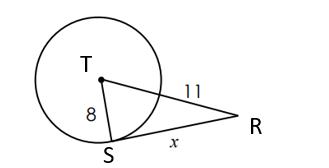SR =

sqrt 297

100

The length of secant RS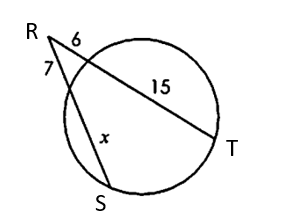RS = 18

100

The length of chord JI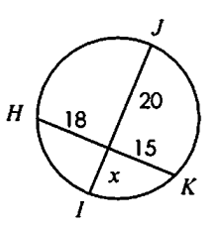JI = 33.5
200

The length of tangent GH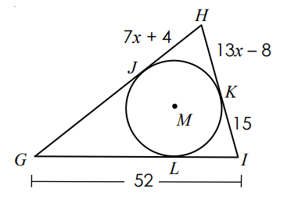GH = 55

200

The length of secant VU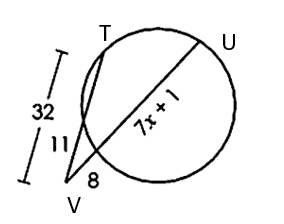VU = 44

200

The length of chord AB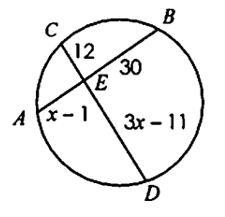AB = 46

300

The TWO possible lengths of tangent RS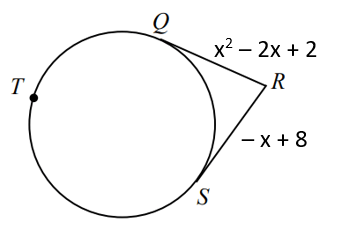RS = 5 or RS = 10

300

The length of secant JL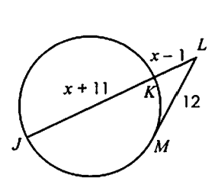JL = 24

300

The TWO possible lengths of chord AD